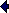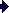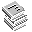Aerostatic thrust bearing fed through an inherent orifice restrictor

Diameter bearing 2R0   10-3 mDiameter orifice 2R1=d_test   10-3 mFilm thickness h0   10-6 m
Supply pressure pS   106 Pa
Ambient pressure pA   106 Pa
Pressure factor β
Coefficient of discharge Cd

Pressure ratio pR/pS
Load capacity F*
Flow rate M   10-6 kg/s
Diameter orifice d   10-3 m
Load capacity F   N
Axial stiffness S=-dF/dh   10 6 N/m
Flow rate Q   l/min

 d_test: The diameter of the inherent orifice restrictor is to be calculated iteratively. In the first step the load capacity and flow are calculated with the outer diameter of the bearing and the diameter of the orifice 2R1=d_test prescribed (equation 12.6 of the book). Next the diameter of the orifice is derived from the calculated flow (equation 12.4) and should be the same as d_test, if not, d_test is to be adapted in this calculator manually.h0: The highest axial bearing stiffness, self-aligning capability and the minimal flow is obtained with the smallest film thickness. The required film thickness depends on bearing deflections and flatness of the bearing surface and is usually related to the bearing size.www.tribology-abc.com